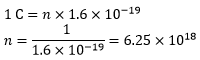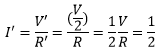• +91 9971497814
• info@interviewmaterial.com

# Chapter 12 – Electricity Interview Questions Answers

### Related Subjects

Question 1 : What does an electric circuit mean?

A continuous closed path made of electric components through which an electric current flows is known as an electric circuit. A simple circuit consists of the following components:
(a) Conductors
(b) Cell
(c) Switch

Question 2 : Define the unit of current.

Answer 2 : The unit of current is ampere. Ampere is defined by the flow of one coulomb of charge per second.

Question 3 : Calculate the number of electrons constituting one coulomb of charge.

The value of the charge of an electron is 1.6 × 10-19 C.
According to charge quantization,
Q = nqe, where n is the number of electrons and qe is the charge of an electron.
Substituting the values in the above equation, the number of electrons in a coulomb of charge can be calculated as follows:Therefore, the number of electrons constituting one coulomb of charge is 6. 25 × 1018.

Question 4 : Name a device that helps to maintain a potential difference across a conductor.

Answer 4 : Battery consisting of one or more electric cells is one of the devices that help to maintain a potential difference across a conductor.

Question 5 : What is meant by saying that the potential difference between two points is 1 V?

Answer 5 : When 1 J of work is done to move a charge of 1 C from one point to another, it is said that the potential difference between two points is 1 V.

Question 6 : How much energy is given to each coulomb of charge passing through a 6 V battery?

We know that the potential difference between two points is given by the equation,
V = W/Q, where,
W is the work done in moving the charge from one point to another
Q is the charge
From the above equation, we can find the energy given to each coulomb as follows:
W = V × Q
Substituting the values in the equation, we get
W = 6V × 1C = 6 J
Hence, 6 J of energy is given to each coulomb of charge passing through a 6 V of battery.

Question 7 : On what factors does the resistance of a conductor depend?

The resistance of the conductor depends on the following factors:
a. Temperature of the conductor
b. Cross-sectional area of the conductor
c. Length of the conductor
d. Nature of the material of the conductor

Question 8 : Will current flow more easily through a thick wire or a thin wire of the same material, when connected to the same source? Why?

Resistance is given by the equation,
R = ρ l/A
where,
ρ is the resistivity of the material of the wire,
l is the length of the wire
A is the area of the cross-section of the wire.
From the equation, it is evident that the area of the cross-section of wire is inversely proportional to the resistance. Therefore, thinner the wire, more the resistance and vice versa. Hence, current flows more easily through a thick wire than a thin wire.

Question 9 : Let the resistance of an electrical component remain constant while the potential difference across the two-

Answer 9 : ends of the component decreases to half of its former value. What change will occur in the current through it?

The change in the current flowing through the electrical component can be determined by Ohm’s Law.
According to Ohm’s Law, the current is given by
I = V/R
Now, the potential difference is reduced to half keeping the resistance constant,
Let the new voltage be V’ = V/2
Let the new resistance be R’ = R and the new amount of current be I’.
The change in the current can be determined using Ohm’s law as follows:Therefore, the current flowing the electrical component is reduced by half.

Question 10 : Why are coils of electric toasters and electric irons made of an alloy rather than a pure metal?

Answer 10 : The melting point of an alloy is much higher than a pure metal because of its high resistivity. At high temperatures, alloys do not melt readily. Therefore, alloys are used in heating appliances such as electric toasters and electric irons.

Todays Deals### Chapter 12 – Electricity Contributorskrishan

Name:
Email:

# Latest News# 9000 interview questions in different categories